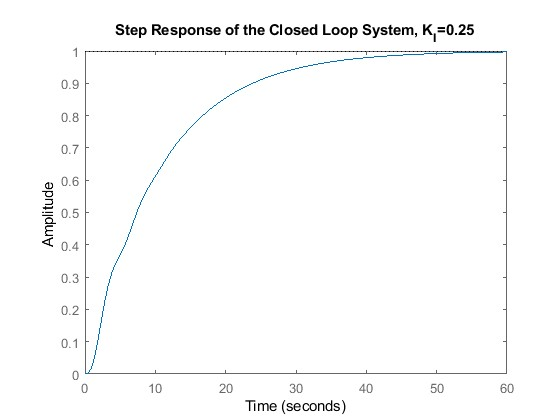# MATLAB control instrumentation

SECTION A: Instrumentation

Part A1: Sensor Selection

The last 4 digits of student id is 2684

Therefore,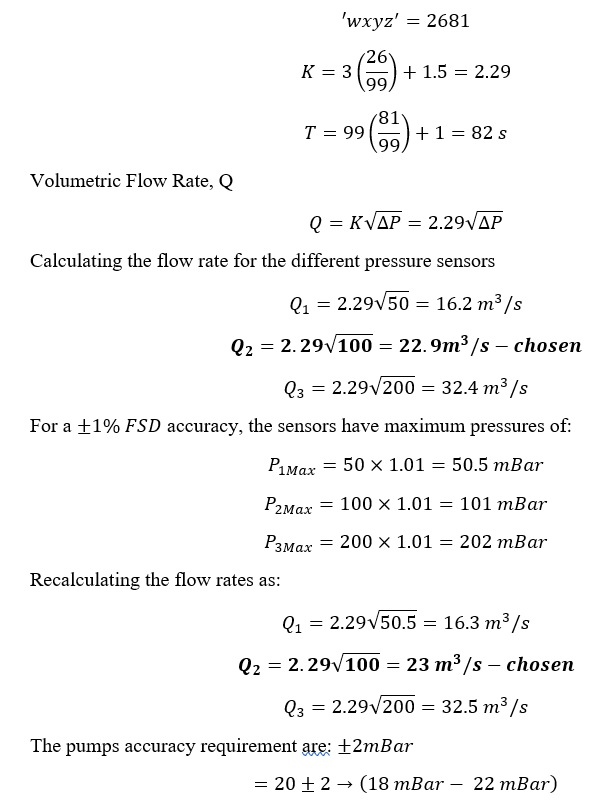The most appropriate sensor is the differential pressure sensor with the range of 0-100mBar as described by the choices above.

Part A2: Transient Response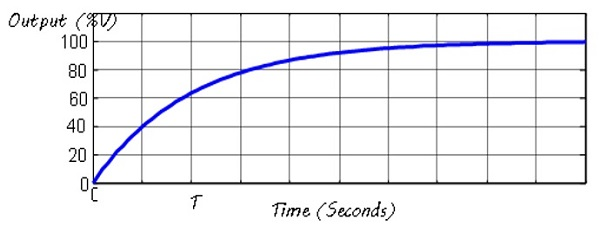After switching the pump “on”, the step response takes T=82 s to reach 63.2% of its steady state value as shown in the figure. This indicates that the time constant of the pump is τ=82 seconds.

The rise time of the system is related to the time constant approximately by:

Tr=2.197 τ

Tr=2.197×82=180.154 s

As the pump is gradually switched on from “off” to “fully on”, the output response goes from transient state to a steady state value of 100 for large values of t. The step response, however, has no overshoot and as can be seen, the system is overdamped.

To calculate the bandwidth, we assume an oscilloscope that follows the following rule:

```{`
Bandwidth ×Rise time=0.35
Bandwidth,BW=0.35/180.154=0.00194 dB
20 logBW=0.00194
logBW=0.00194/20=0.000097
`}```

Part A3: Filter Design

Using an RC filter design

Let:

C=10uF

Corner frequency is at two octaves above the bandwidth of the system at unity gain, K=1

Therefore: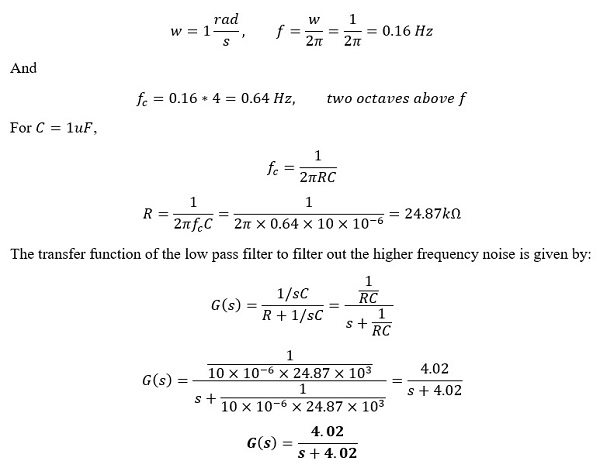SECTION B: Control

Part B1

Modelling

G(s)=Y(s)/U(s) =A/(s2+As+A.B)

The last 4 digits of student id is 2684

Therefore,

```{`
'wxyz^'=2681
A=0.9(26/99)+0.5=0.74
B=2(81/99)+1=2.64
A=0.74,B=2.64
`}```

The underdamped second order system is given by:

G(s)=0.74/(s2+0.74s+0.74(2.64))

=0.74/(s2+0.74s+1.9536)

Generally, the second-order transfer function takes the form of:

G(s)=(w02)/s2+2ζwo s+w20

Where w0 is the natural frequency, ζ is the damping ratio.

Therefore, by comparison of the 2 equations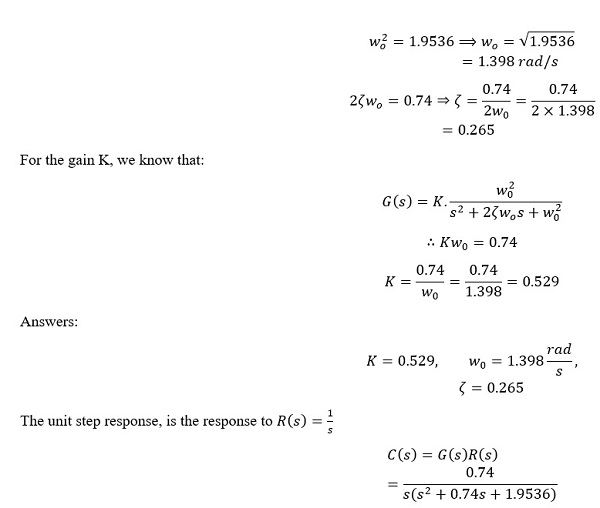Plotting in MATLAB

Part B2: Control

Part (i)

Finding the closed loop system transfer function

Forward path/Open loop transfer function

Y(s)/E(s) =KI/s (G(s))

=(0.74 KI)/(s(s2+0.74s+1.9536))

Closed loop transfer function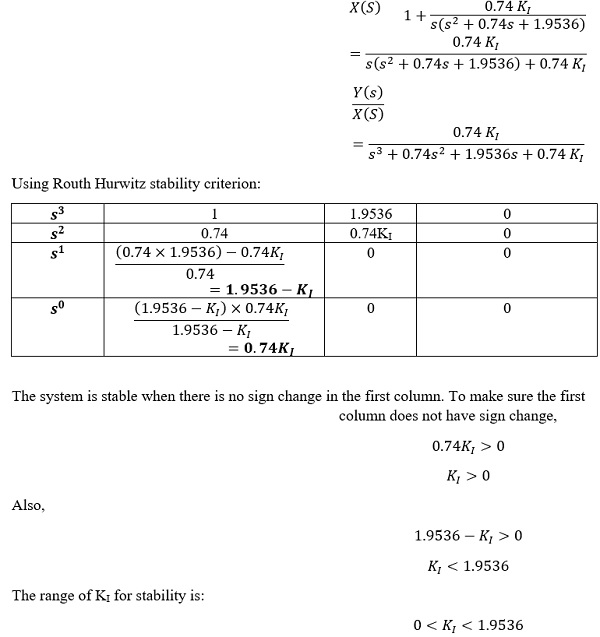```{`
clear
clc
s=tf("s");
G=0.74/(s2+0.74*s+1.9536);
figure (1)
step(G)
title("Step Response of the Open Loop System")
`}```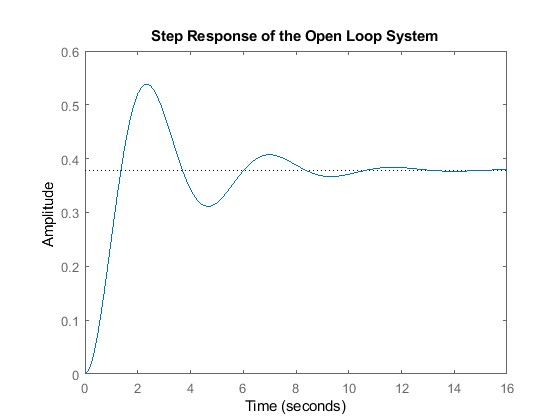Part (ii)

Choosing a KI of 0.25 which is in the range of , the step input response is obtained using MATLAB.

```{`
%Let Ki=0.25 since for stability 0< Ki <1.9536
Integral_Controller=0.25/s;
G1=Integral_Controller*G;
T=feedback(G1,1);
StableOrNot=isstable(T)
figure(2)
step(T)
title("Step Response of the Closed Loop System, K_I=0.25")
`}```

StableOrNot =

logical

1